• Call Now

1800-102-2727•

Introduction to EMI,Faraday’s laws, Practice problems, FAQs

You copy a file on your computer; the information gets stored in its hard disk. You go to the ATM machine, you swipe your debit card, the machine makes a buzzing noise and then dispenses cash. The science behind these is a phenomenon called “electromagnetic induction”. A scientist named Faraday, around 1831, conducted an experiment with a magnet and a conductor coil. He noticed that when the magnet was moved keeping the coil stationary, a current was produced in the coil. Current was also produced if the coil was rotated about its diameter keeping the magnet stationary. From this, he was able to conclude that electricity and magnetism share a close relation. Now how does this relate to a debit card, swiped in a machine? The machine’s wires cause a change in magnetic field which produces a current that relays your card’s details to the machine. Hard disks contain a spinning disk with a magnetic layer and store information in binary language (0 and 1). Many equipments like magnetic brakes, induction cookers, generators work on the principle of electromagnetic induction.

• Magnetic flux
• Electromagnetic induction
• Faraday’s laws of electromagnetic induction
• Induced charge
• Practice problems
• FAQs

## Magnetic flux

Magnetic lines of force produced by a magnet start from its North (N) pole and end in its South(S) pole. They always form closed loops.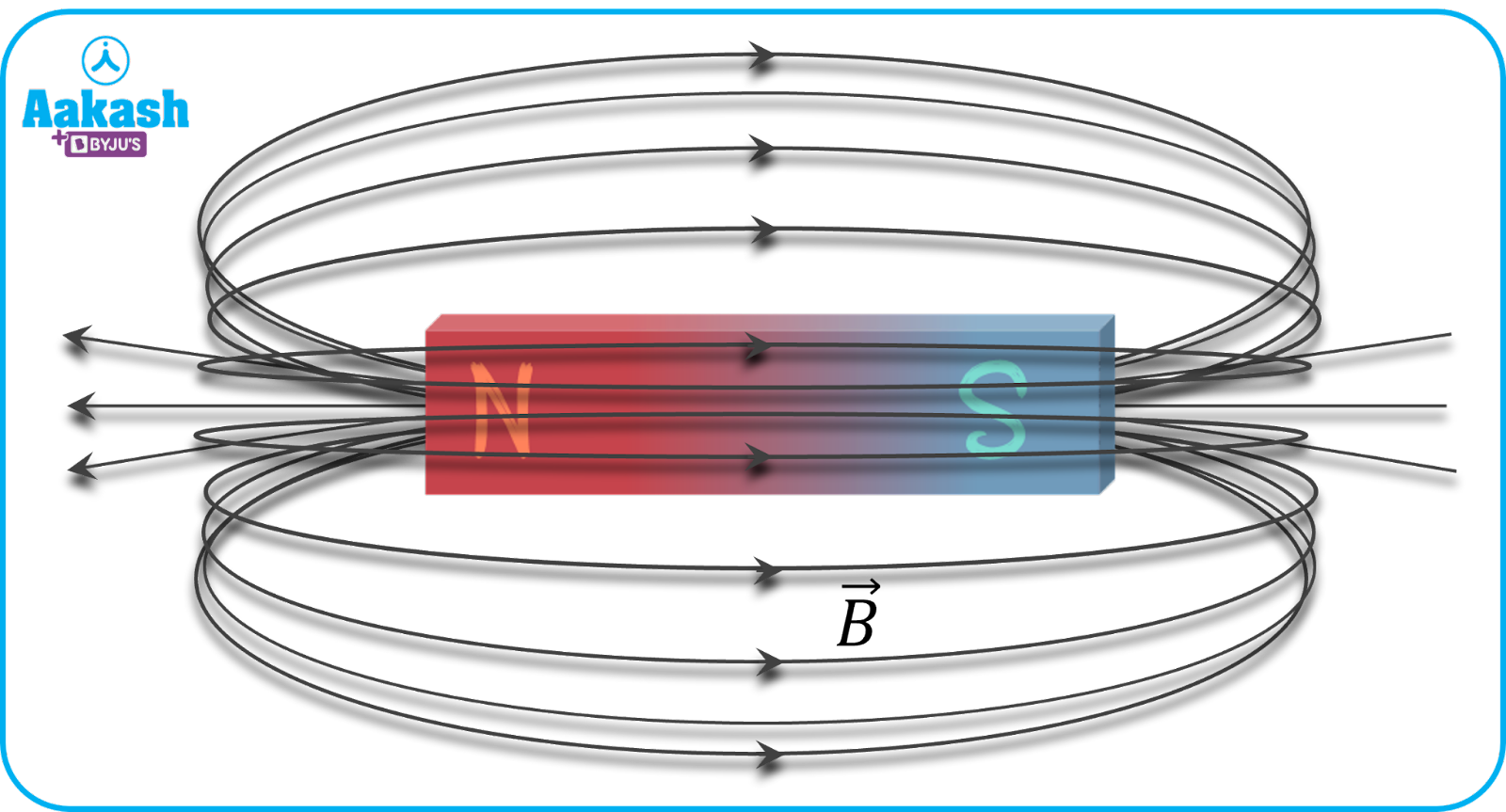Magnetic lines of force

The number of magnetic lines of force crossing a given area held perpendicular to the surface (i.e. the area vector) is called magnetic flux (or, the most used quantity, magnetic flux density when calculated over a unit area). Let $\stackrel{\to }{B}$ indicates the value of magnetic field passing through a surface whose area vector is $\stackrel{\to }{A}$. Then, the magnetic flux () can be written as the dot product between $\stackrel{\to }{B}$ and A. i.e =$\stackrel{\to }{B}$.$\stackrel{\to }{A}$=$\stackrel{\to }{B}$ A cos where is the angle between $\stackrel{\to }{B}$ and A.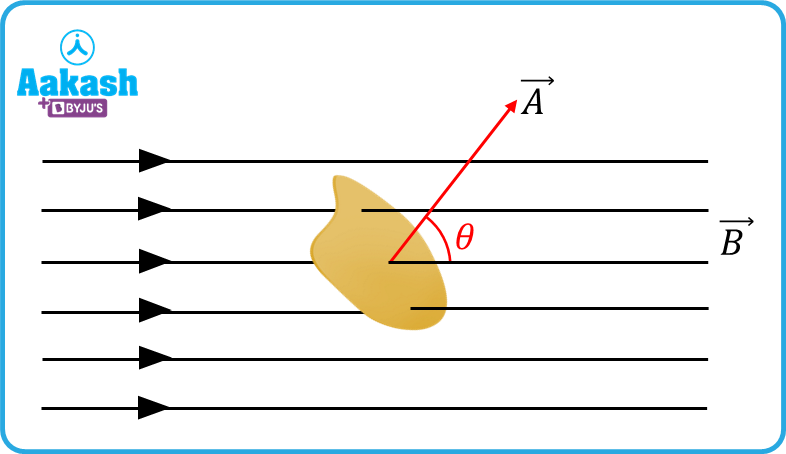Magnetic flux is a scalar quantity. Its SI unit is Weber (Wb) and its CGS unit is Maxwell.

## Specific cases of magnetic flux:

(i) When the coil is held perpendicular to the direction of $\stackrel{\to }{B}$, angle between $\stackrel{\to }{B}$ and A is =00. Then flux =B A cos $\theta$=BA.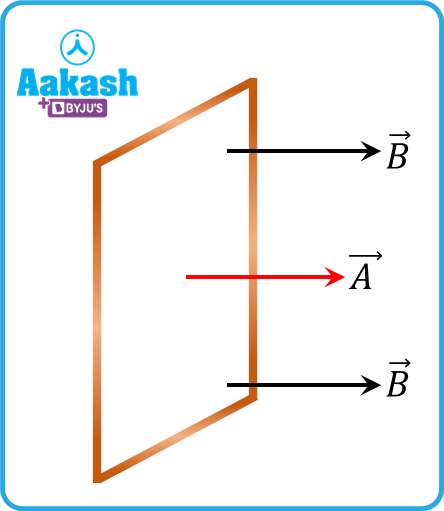(ii) When the coil is held parallel to the direction of $\stackrel{\to }{B}$, angle between $\stackrel{\to }{B}$ and $\stackrel{\to }{A}$ is =900. Then flux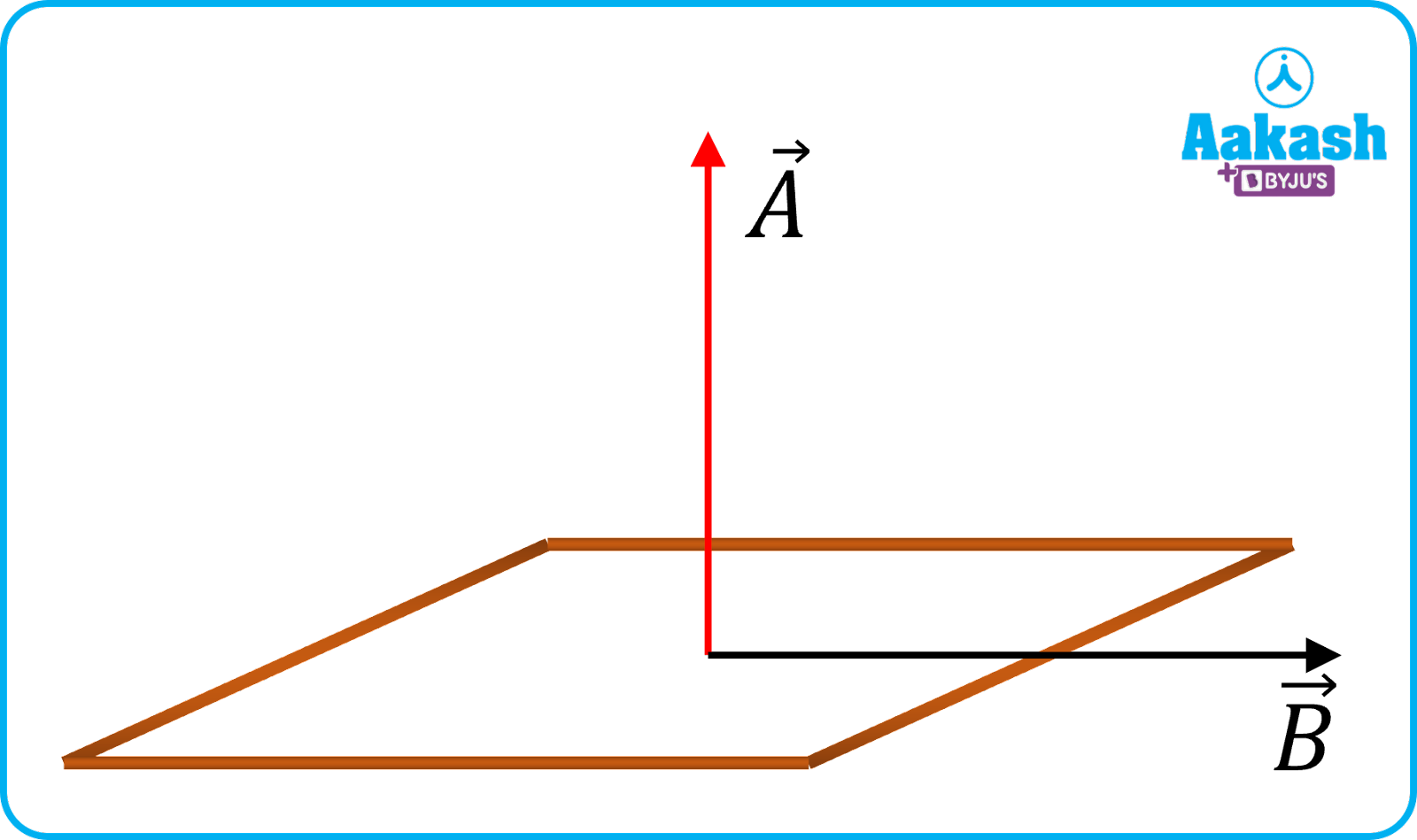(iii) When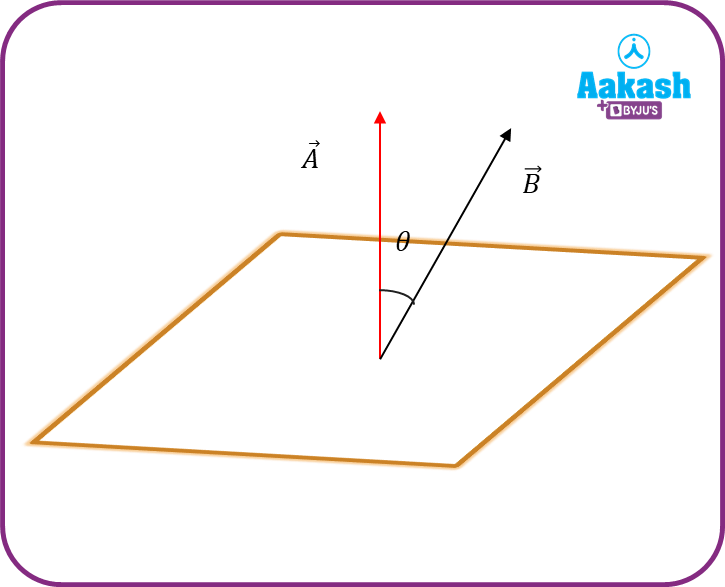(iv) When(v) When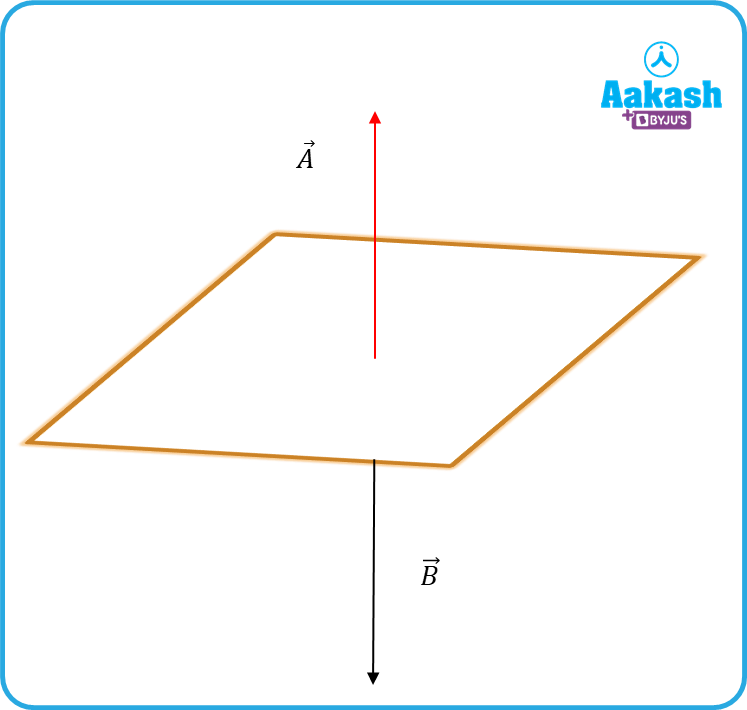## Electromagnetic Induction

Let us take a coil connected to a galvanometer. A Galvanometer is a device which can detect currents. A bar magnet, which produces magnetic flux, is initially held stationary. Since there is no change in magnetic flux, the galvanometer shows no deflection. But when the bar magnet is moved relative to the coil, then a small deflection is observed in the galvanometer. Whenever the magnetic flux linked with a coil changes, then an emf (electromotive force) is induced in it. The emf produces an induced current in the coil if the circuit is made closed. The induced emf and current are further explained by Faraday’s laws.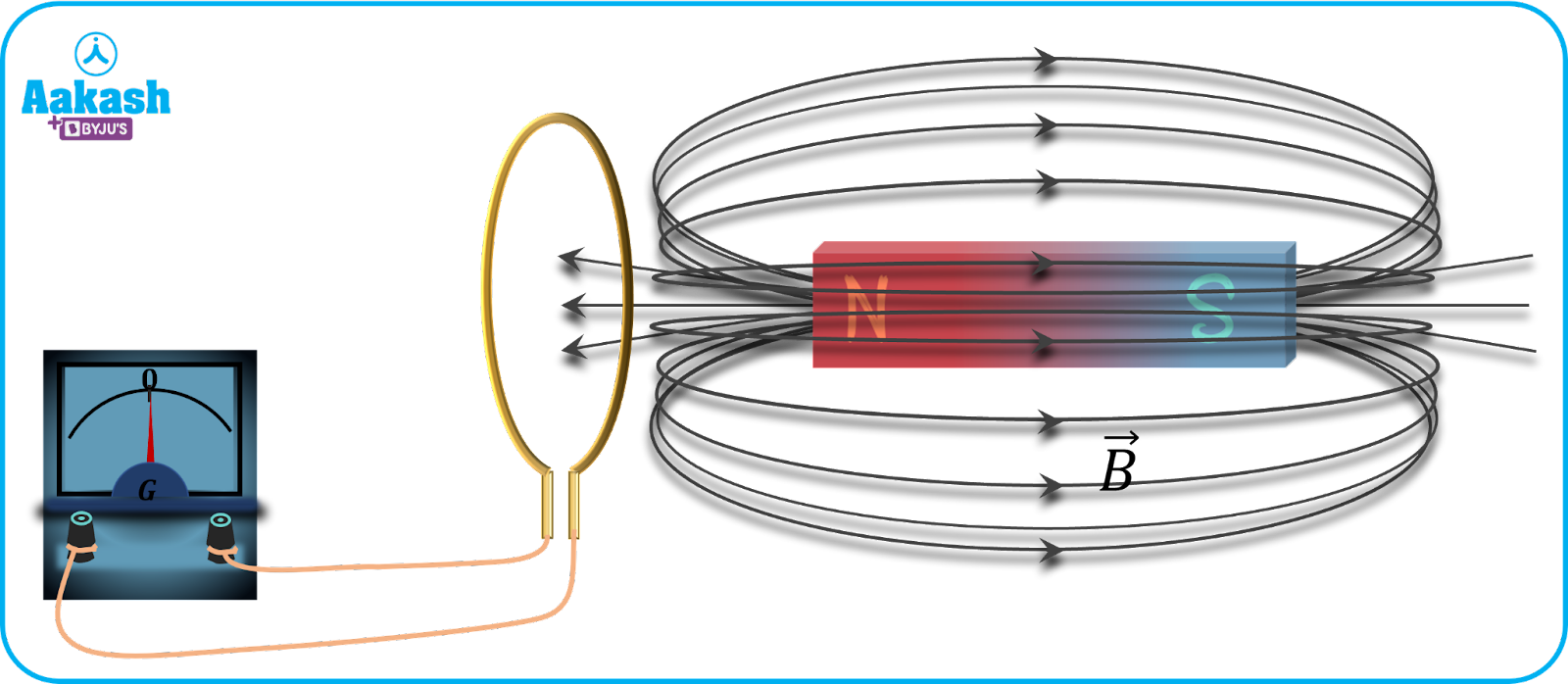(i) Faraday’s first law of electromagnetic induction
Whenever the magnetic flux linked with a coil changes, an emf is induced in the coil.

(ii) Faraday’s second law of electromagnetic induction
The magnitude of the induced emf is equal to the rate of change of magnetic flux associated with the coil.

$e=-\frac{d\varphi }{dt}---\left(i\right)\phantom{\rule{0ex}{0ex}}\left|e\right|=\left|\frac{d\varphi }{dt}\right|$

Let, d is the change in magnetic flux associated with the coil in time interval dt. Let A indicates the cross sectional area of the conductor coil and B be the magnetic field. If N indicates the number of turns in the coil, then the flux associated with the coil,

Substituting equation (ii) in equation (i), we get

$e=-\frac{d\left(NBAcos\theta \right)}{dt}--\left(iii\right)$

Now, here the coil is rotated by an angle, keeping the magnetic field, B stationary, then =⍵t

where, ⍵ indicates the angular velocity of the coil.

Equation (iii) becomes

If the circuit is closed, the induced current i produced in the coil, $i=\frac{e}{R}$

Here, R indicates the resistance of the coil.

$i=NB⍵Asin⍵tR={i}_{0}sin⍵t$

${i}_{0}=NBA⍵R$ indicates the peak value of current induced in the coil.

In the above case, B indicates a uniform magnetic field i.e., the magnitude of the magnetic field is the same at all points. When the magnetic field also varies with time, equation (iii) becomes

$\frac{dB}{dt}$indicates change in magnetic field with respect to time.

Induced charge

As a result of current induced in the circuit, charge also starts flowing in it. Let dq be the charge flowing in time dt. Then, dq=idt,

$i=\frac{e}{R},e=-\frac{d\varphi }{dt}⇒i=-\frac{1}{R}\frac{d\varphi }{dt}$

Therefore, charge flown becomes $dq=-\frac{1}{R}\frac{d\varphi }{dt}.dt=-\frac{1}{R}d\varphi dq=-\frac{d\varphi }{R}$

If a coil is having N turns, of cross sectional area A is rotated from an angle i to an angle f in a magnetic field B, the charge flown becomes

$q=\frac{NBA\left(cos{\theta }_{i}-cos{\theta }_{f}\right)}{R}$

Video explanation

Practice problems

Q. 1 coil having cross-sectional area 5 m2, resistance 10 and number of turns 200 is held perpendicular to a uniform magnetic field of strength 0.4 T. The coil is now turned through 1800 in a time of 1 s. Find,

(i) the peak induced emf

(ii) peak induced current

(iii) total charge that has flown through the coil?

A. Given, B

Change in flux of the coil

(i) peak induced emf,

(ii) peak induced current,

(iii) Total charge flown

Q2. The magnetic flux across a loop of resistance 10 varies as a function of time given by =(5t2-4t+1) Wb. How much current is induced in the loop after 0.2 s ?

(a) 0.4 A (b) 0.2 A (c) 0.04 A (d) 0.02 A

A. b

$e=-\frac{d\varphi }{dt}=-\frac{d}{dt}\left(5{t}^{2}-4t+1\right)=-\left(10t-4\right)$

At t=0.2 s, induced emf becomes -

Now induced current

Q3. A coil having an area of A0 is placed in a magnetic field which changes from B0 to 4B0 in time interval t. The emf induced in the coil will be

A. a

The magnitude of induced emf $e=-\frac{d\varphi }{dt}=-\frac{d\left(BA\right)}{dt}$

$=-{A}_{0}\left(\frac{{4B}_{0}-{B}_{0}}{t}\right)=-\frac{3{A}_{0}{B}_{0}}{t}$

So the magnitude is $\frac{3{A}_{0}{B}_{0}}{t}$

Q4. A ring is having a diameter of 20 cm having a resistance of How much charge will flow through the ring, if it is initially held perpendicular to the magnetic field having an intensity of 2 T and then rotated to bring it parallel to the direction of the field ?

A.b

Given,

B=2 T

Let dq be the charge flown. Then $dq=\frac{d\varphi }{R}$

Given, ${\theta }_{i}={0}^{0},{\theta }_{f}={90}^{0}$

=BA(cosi-cosf)=2 (0.1)2(cos0-cos 90)=0.0628 Wb

FAQs

Q. What items are needed to conduct Faraday's experiment?
A.
Bar magnets, copper wire and a galvanometer to detect the voltage or current are needed in order to conduct Faraday's experiment.

Q. How is Faraday’s law related to Lenz’s law?
A.
The negative sign in Faraday’s law indicates that the induced emf opposes the cause which produces it. This is in accordance with Lenz’s law.

Q. Why is Faraday’s law important?
A.
Faraday’s law explains that changing magnetic flux induces an emf in the coil which causes current to flow in the circuit. This is made to use in electrical devices like motors.

Q. How does Faraday’s law work?
A.
It states that the absolute value of electric field circulating along a coil is equal to the rate of change of magnetic flux through the area that is enclosed by the loop.Talk to our expert
Resend OTP Timer =
By submitting up, I agree to receive all the Whatsapp communication on my registered number and Aakash terms and conditions and privacy policy